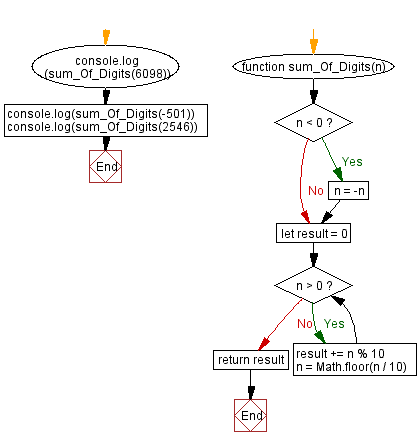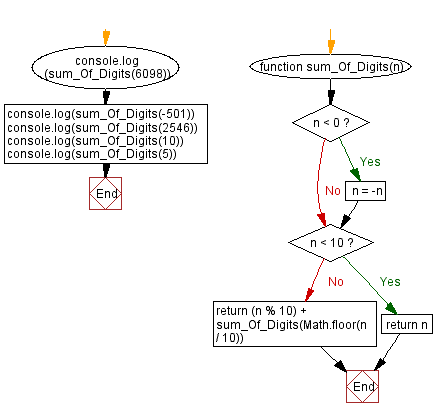# JavaScript: Sum of the digits of a number

## JavaScript Math: Exercise-68 with Solution

Write a JavaScript program to calculate the sum of a given number's digits.

In mathematics, the digit sum of a natural number in a given number base is the sum of all its digits. For example, the digit sum of the decimal number 6098 would be 6+0+9+8=23.

Sample Data:
6098 -> 23
-501 -> 6
2546 -> 17

Sample Solution-1:

HTML Code:

``````<!DOCTYPE html>
<html>
<meta charset="utf-8">
<title>JavaScript function to sum of the digits of a number</title>
<body>

</body>
</html>
```
```

JavaScript Code:

``````function sum_Of_Digits(n) {
if (n < 0) n = -n
let result = 0

while (n > 0)
{
result += n % 10
n = Math.floor(n / 10)
}

return result
}
console.log(sum_Of_Digits(6098))
console.log(sum_Of_Digits(-501))
console.log(sum_Of_Digits(2546))
```
```

Sample Output:

```23
6
17
```

Flowchart:Live Demo:

See the Pen javascript-math-exercise-68 by w3resource (@w3resource) on CodePen.

Sample Solution-2:

JavaScript Code:

``````function sum_Of_Digits(n) {
if (n < 0) n = -n
if (n < 10) return n
return (n % 10) + sum_Of_Digits(Math.floor(n / 10))
}
console.log(sum_Of_Digits(6098))
console.log(sum_Of_Digits(-501))
console.log(sum_Of_Digits(2546))
console.log(sum_Of_Digits(10))
console.log(sum_Of_Digits(5))
```
```

Sample Output:

```23
6
17
1
5
```

Flowchart:Live Demo:

See the Pen javascript-math-exercise-68-1 by w3resource (@w3resource) on CodePen.

Improve this sample solution and post your code through Disqus

What is the difficulty level of this exercise?

Test your Programming skills with w3resource's quiz.

﻿